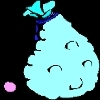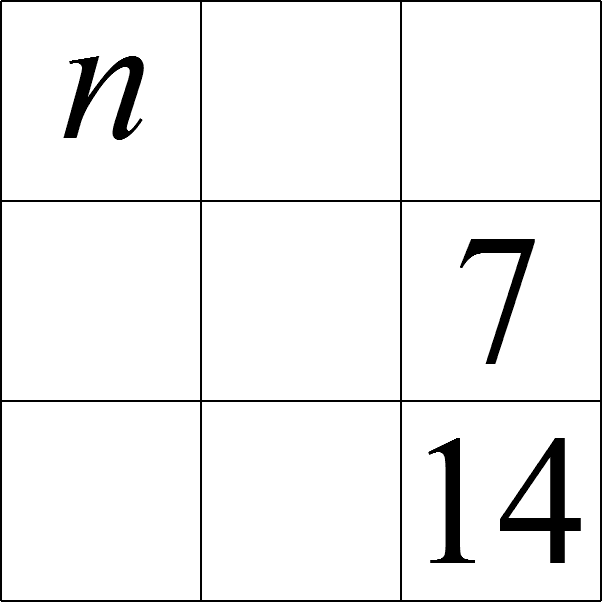#### You may also like### Inequalities

A bag contains 12 marbles. There are more red than green but green and blue together exceed the reds. The total of yellow and green marbles is more than the total of red and blue. How many of each colour there are in the bag?### What's it Worth?

There are lots of different methods to find out what the shapes are worth - how many can you find?### Turnips

Baldrick could buy 6 parsnips and 7 turnips, or 8 parsnips and 4 turnips. How many parsnips could he buy?

# Magic 7

##### Age 11 to 14 Short Challenge Level:In this magic square, which uses all the integers from $7$ to $15$, each of the rows, columns and the two main diagonals has the same total.

Which number replaces $n$ in the completed square?

If you liked this problem, here is an NRICH task that challenges you to use similar mathematical ideas.

This problem is taken from the UKMT Mathematical Challenges.
You can find more short problems, arranged by curriculum topic, in our short problems collection.